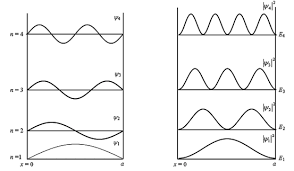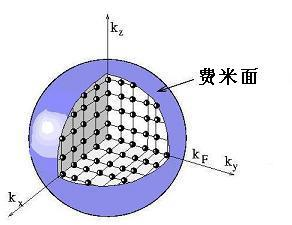# 自由电子模型

## 一维情况下的能级和轨道密度（一维无限深势阱）

• 质量为 m 的电子被无限高势垒限制在长度为 L 的直线上
• 引入薛定谔方程： $\hat{H} \psi = \varepsilon \psi$
• 电子的波函数 $\psi_n(x)$ 是方程的解
• $\hat{H} = \frac{p^2}{2m}=-\frac{\hbar^2}{2m} \frac{\dif^2}{\dif x^2}$
• 略去 $\hat{H}$ 中的势能
• $p$ 是动量算子 $p = - i \hbar \frac{\dif}{\dif x}$• 以同一量子数 $n$ 标记的一对轨道能容纳两个电子，它们自旋相反，能量相同
• 相同能量的轨道可以不止一个，具有相同能量的轨道数目称为 简并度
• 电子先从低能级轨道开始填充

$\varepsilon_F$ 定义为费米能，是基态下最高被充满能级的能量，对应能级为费米能级。我们注意到 $\frac{N}{L}$ 的量纲为长度的倒数，有点像倒易点阵中的矢量，同时其物理意义是“密度”，意味着费米能级不随物体大小而改变。

## 温度对费米-狄拉克分布的影响## 三维情况下的自由电子气1. 任意两个电子可区分，即不存在两个电子量子数相同